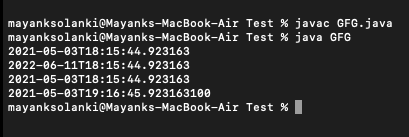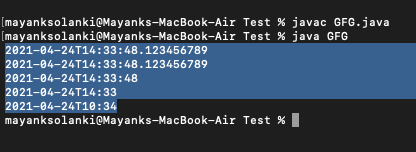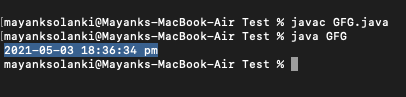# java.time.LocalDateTime Class in Java

• Last Updated : 26 May, 2021

java.time.LocalDateTime class, introduced in Java 8, represents a local date-time object without timezone information. The LocalDateTime class in Java is an immutable date-time object that represents a date in the yyyy-MM-dd-HH-mm-ss.zzz format. It implements the ChronoLocalDateTime interface and inherits the object class.

Wherever we need to represent time without a timezone reference, we can use the LocalDateTime instances. LocalDateTime, for example, can be used to start batch jobs in any application. Jobs will be run at a fixed time in the timezone in which the server is located. Note LocalDateTime instances are immutable and thread.

Attention reader! Don’t stop learning now. Get hold of all the important Java Foundation and Collections concepts with the Fundamentals of Java and Java Collections Course at a student-friendly price and become industry ready. To complete your preparation from learning a language to DS Algo and many more,  please refer Complete Interview Preparation Course.

Syntax: Class declaration

public final class LocalDateTime

extends Object

Methods of this class are as follows:

Some more methods to modify local time are as follows  in LocalDateTime can be used to get to a new localdatetime instance relative to an existing localdatetime instance. They are namely as follows:

plusYears(), plusMonths(), plusDays(), plusHours(), plusMinutes(), plusSeconds(), plusNanos(), minusYears(), minusMonths(), minusDays(), minusHours(), minusMinutes(), minusSeconds(), minusNanos()

Examples 1:

## Java

 `// Java Program to illustrate LocalDateTime Class of java.time package ` `// Importing LocalDateTime class from java.time package``import` `java.time.LocalDateTime;` `// Main class for LocalDateTime``public` `class` `GFG {` `    ``// Main driver method``    ``public` `static` `void` `main(String[] args)``    ``{``        ``// Creating an object of LocalDateTime class``        ``// in the main() method``        ``LocalDateTime now = LocalDateTime.now();` `        ``// Print statement``        ``System.out.println(now);` `        ``// Adding 1 year, 1 month, 1 week and 1 day``        ``LocalDateTime localDateTime1 = now.plusYears(``1``)``                                           ``.plusMonths(``1``)``                                           ``.plusWeeks(``1``)``                                           ``.plusDays(``1``);``        ``// Print statement``        ``System.out.println(localDateTime1);` `        ``// Subtracting 1 year, 1 month, 1 week and 1 day``        ``LocalDateTime localDateTime2``            ``= localDateTime1.minusYears(``1``)``                  ``.minusMonths(``1``)``                  ``.minusWeeks(``1``)``                  ``.minusDays(``1``);``        ``// Print statement``        ``System.out.println(localDateTime2);` `        ``// Adding 1 hour, 1 minute, 1 second and 100``        ``// nanoseconds``        ``LocalDateTime localDateTime3``            ``= localDateTime2.plusHours(``1``)``                  ``.plusMinutes(``1``)``                  ``.plusSeconds(``1``)``                  ``.plusNanos(``100``);``        ``// Print statement``        ``System.out.println(localDateTime3);` `        ``// Subtracting 1 hour, 1 minute, 1 second and 100``        ``// nanoseconds``        ``LocalDateTime localDateTime4``            ``= localDateTime3.minusHours(``1``)``                  ``.minusMinutes(``1``)``                  ``.minusSeconds(``1``)``                  ``.minusNanos(``100``);``        ``// Print statement``        ``System.out.println(localDateTime4);``    ``}``}`

Output:Example 2: Creating a specified time

## Java

 `// Java Program to illustrate LocalDateTime Class``// of java.time package by creating specific time` `// Importing required classes from resp packages``import` `java.time.*;``import` `java.time.format.*;` `// main class``class` `GFG {` `    ``// Main driver method``    ``public` `static` `void` `main(String[] args)``    ``{` `        ``// Milliseconds``        ``LocalDateTime localDateTime1 = LocalDateTime.of(``            ``2021``, ``04``, ``24``, ``14``, ``33``, ``48``, ``123456789``);``        ``// Print statement``        ``System.out.println(localDateTime1);` `        ``// Month``        ``LocalDateTime localDateTime2 = LocalDateTime.of(``            ``2021``, Month.APRIL, ``24``, ``14``, ``33``, ``48``, ``123456789``);``        ``// Print statement``        ``System.out.println(localDateTime2);` `        ``// Seconds``        ``LocalDateTime localDateTime3 = LocalDateTime.of(``            ``2021``, Month.APRIL, ``24``, ``14``, ``33``, ``48``);``        ``// Print statement``        ``System.out.println(localDateTime3);` `        ``// Minutes``        ``LocalDateTime localDateTime4 = LocalDateTime.of(``            ``2021``, Month.APRIL, ``24``, ``14``, ``33``);``        ``// Print statement``        ``System.out.println(localDateTime4);` `        ``// Local date + Local time``        ``LocalDate date = LocalDate.of(``2021``, ``04``, ``24``);``        ``LocalTime time = LocalTime.of(``10``, ``34``);` `        ``LocalDateTime localDateTime5``            ``= LocalDateTime.of(date, time);``        ``// Print statement``        ``System.out.println(localDateTime5);``    ``}``}`

Output:Example 3: Format LocalDateTime to string

To format a local time to the desired string representation, use the LocalDateTime.format(DateTimeFormatter) method.

## Java

 `// Java Program to illustrate LocalDateTime Class by``// Formatting LocalDateTime to string` `// Importing all classes from java.time package``import` `java.time.LocalDateTime;``import` `java.time.format.*;``import` `java.util.*;` `// Main class``class` `GFG {` `    ``// Main driver method``    ``public` `static` `void` `main(String[] args)``    ``{` `        ``// Creating an object of DateTimeFormatter class``        ``DateTimeFormatter formatter``            ``= DateTimeFormatter.ofPattern(``                ``"yyyy-MM-dd HH:mm:ss a"``);` `        ``// Creating an object of LocalDateTime class``        ``// and getting local date and time using now()``        ``// method``        ``LocalDateTime now = LocalDateTime.now();` `        ``// Formatting LocalDateTime to string``        ``String dateTimeString = now.format(formatter);` `        ``// Print and Display``        ``System.out.println(dateTimeString);``    ``}``}`

Output:Note: In order to parse a string to LocalDateTime, convert time in a string to a local time instance, the LocalDateTime class has two overloaded parse() methods.

• parse(CharSequence text)
• parse(CharSequence text, DateTimeFormatter formatter)

My Personal Notes arrow_drop_up